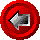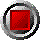HW_cap_var_strike() and HW_floor_var_strike() functionsHW_cap_var_strike() and HW_floor_var_strike() functions

HW_cap_var_strike(argument list…)

HW_floor_var_strike(argument list…)

These functions return the value of caps and floors with varying principal amounts and strikes, using the Hull-White analytic model. The functions use the following arguments:

 Argument Description Restrictions Valuation_Date valuation date (e.g. today) valid Excel date number Principal_Dates array of principal dates strictly ascending order Principal_Amounts array of principal amounts (last element is unused) >= 0 Caplet_Strikes or Floorlet_Strikes array of option strike rates in decimal form (e.g. six percent entered as 0.06) corresponding to the above arrays > 0 Year_Basis year basis used in determining payments 360 or 365 Zero_Dates array of zero coupon curve dates strictly ascending order The first date of this array must be Valuation_Date Zero_Rates array of continuously compounded riskless rates in decimal form (e.g. six percent entered as 0.06) corresponding to Zero_Dates > 0 Short_Rate_Vol annual standard deviation of the short rate of interest, in decimal form > 0 Reversion_Rate mean reversion rate of the short rate of interest, in decimal form >= 0

© 1995-98 Leap of Faith Research Inc.